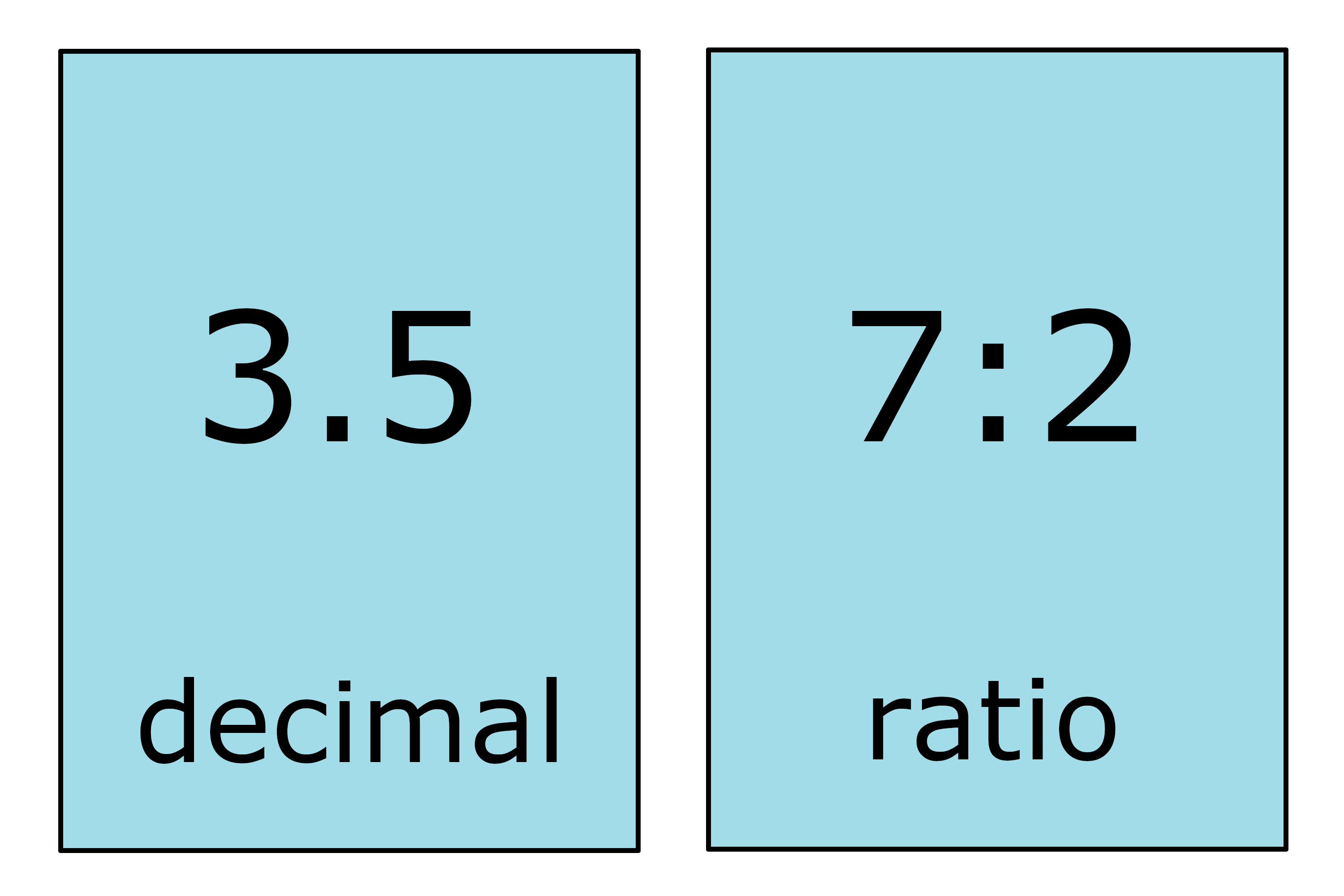# Decimal to Ratio Calculator

Enter a decimal below to convert it to a ratio.

## Ratio:

4 : 5

### Steps to Convert a Decimal to a Ratio

Step One: Convert the decimal to a fraction
0.8=45
Step Two: Rewrite the fraction as a ratio
45=4:5

## How to Convert a Decimal to a Ratio

You might come across a need to express a decimal as a ratio, and that’s possible to do in a few easy steps.

### Step One: Express the Decimal to a Fraction

The first step in converting a decimal to a ratio is to express the decimal as a fraction. You can use a decimal to fraction calculator to do that, but you can also do it yourself.

To convert a decimal to a fraction, start by placing the decimal as the numerator over the denominator of 1. Then, continue multiplying both the numerator and denominator by 10 until the numerator is a whole number.

You can optionally simplify the fraction at this point.

### Step Two: Rewrite the Fraction as a Ratio

The second step in converting a decimal to a ratio is to rewrite the fraction in ratio form. To do so, place the numerator in front of the denominator and separate them with a colon (:) symbol.

For example, convert 3.5 to a ratio.

Start by converting 3.5 to a fraction.
3.5 = 3.51
3.5 = (3.5 × 10)(1 × 10)
3.5 = 3510

Then simplify.
3510 = (35 ÷ 5)(10 ÷ 5)
3510 = 72

Finally, rewrite as a ratio.
72 = 7:2

Thus, the ratio is 7:2You’ll probably also find our ratio to decimal calculator useful.